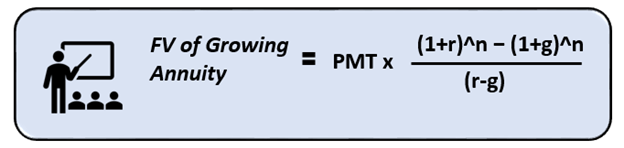# FV of Annuity

## What is Future Value of Annuity?

An annuity creates a guaranteed yearly return on investment where payments come in consistently at the end of each period. The future value of an annuity (FV of Annuity) computation shows the future worth of payments from an annuity based on a steady rate of return. The future value of an annuity is the worth of a cluster of recurring disbursements at a certain future date. It assumes a certain rate of return, called the discount rate—the greater the discount rate, the bigger the future value of the annuity and vice versa.

Summary
• The future value of an annuity (FV of Annuity) is a total of the worth of annuity disbursements at a particular date in future.
• It is the amount of money an annuity will generate on a future date. It utilises the concept of the time value of money.
• Knowledge of the FV of an annuity can be useful for planning retirement or any other aspect major event needing a lot of money.

## What is Future Value of an Annuity?

It is the application of the time value of money concept to an annuity payment/ receipt. Since in the future annuity receipts will not be what it is worth today. An annuity by nature spreads a lump sum future receipt/ payment into a series of annual payments spread out over the annuity period. Thus, it is essentially used by an investor to know real returns from an annuity.

An example of an Annuity Fund would be a scholarship program funded by a scientist’s legacy. The scientist’s legacy is invested to generate annual returns. The annual return is used to pay for a scholarship of young scientists. To know exactly how much funds such a corpus will generate in future, the concept of FV of Annuity is used.

## How is FV of annuity computed?

This is the formula for determining the future value of an annuity:Here,

• PMT = the value of each annuity payment
• r = the interest rate
• n = the number of periods over which payments will be made

In case it is a growing annuity, where disbursements grow over time, the formula for computing future value of such a growing annuity will be:Here,

• PMT = the value of each annuity payment
• r = the interest rate
• n = the number of periods over which payments will be made
• g= the growth rate

Growing annuity assumes that the earnings will be ploughed back to get higher annual returns in future.

Both the above-mentioned calculations are used by firms and individuals to regulate cash flows related to their financial products.

## Example of Computation-

Suppose Ironman is going to get a US\$10,000 annuity payment each year for the next five years, at an 8% rate of return. If this data is plugged into the FV of Annuity formula, like:

P = 10,000 x {[(1 + 0.08) ^ 5 – 1] / 0.08}

After doing the math, Ironman gets the future value of annuity receipts as US\$45,061.12. Now he can use this number while looking for finance in the future to continue with inventions in his lab.

In case it is a growing annuity, where the amount earned is reinvested constantly, and the growth rate is 3%. Then computation will be as follows-

P = 10,000 x {[(1 + 0.08) ^ 5 – (1+0.03) ^5] / (0.08-0.03)}

The resultant future value of annual receipt of US\$46,996.03. There is a slight increase due to the compounding on reinvested returns.

## How can the Future Value of an Annuity be Analysed?

In case the annuity is regarding outflows, the Future value calculated will work as an analytical tool used to estimate the total charge of instalments. Firms can use it to analyse investments that need annual deposits, and the firm wishes to forecast potential return.

It is widely used to compute the future value of funds used for retirement planning. Retirement annuity funds lure investors by promising them a fixed stream of earnings over time, often concluding by the death of the individual. Retired investors are offered multiple options, from long-term deposits to immediate payout funds. The real appealing factor is the consistent payout that investors look out for. To know the real worth of these future receipts, they can use the concept of FV of Annuity and assess the financial reality. It is essential to know the best financial option available to them.

Average investors can also use this concept to become aware of how their investment is faring over time. This will enable them to correctly compare investment prospects.

The difference in the type of annuity can also make a significant difference in the future returns on investment. Compounding and reinvestment make a huge difference in the results. Even the time period for which the amount is being invested plays an important role in determining the return.

Considering the various factors taken into consideration for the calculation of the Future Value of an Annuity helps evaluate the cash worth of an investment over time. It assists in determining how much a series of annuity outflows will be worth at a future date, given a particular interest rate is applied to it.

##### Top ASX Listed Companies

Definition:
We use cookies to ensure that we give you the best experience on our website. If you continue to use this site we will assume that you are happy with it. OK